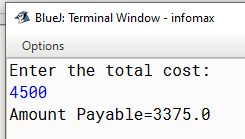# Write a java program to input the total cost and compute and display the amount to be paid by the customer after availing the discount

A cloth showroom has announced the following festival discounts on the purchase of items, based on the total cost of the items purchased:

 Total Cost Discount (in Percentage) Less than ₹ 2000 5% ₹ 2001 to ₹ 5000 25% ₹ 5001 to ₹ 10000 35% Above ₹ 10000 50%

Write a program to input the total cost and compute and display the amount to be paid by the customer after availing the discount.

`Solution`

``````import java.util.*;
class Discount
{
public static void main(String arr[])
{
int tc;
float dis,r,ap;
Scanner sc=new Scanner(System.in);
System.out.println("Enter the total cost:");
tc=sc.nextInt();
if (tc<=2000)
r=5;
else if (tc<=5000)
r=25;
else if (tc<=10000)
r=35;
else
r=50;
dis=r/100*tc;
ap=tc-dis;
System.out.println("Amount Payable="+ap);
}
}``````
`Output`